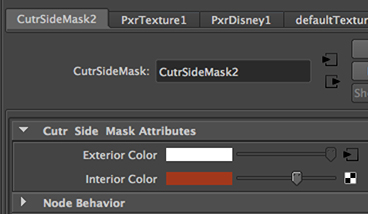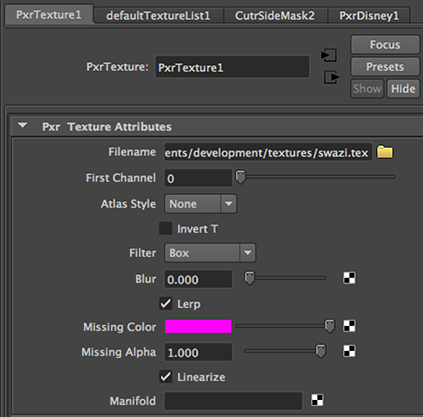### RixPatternsAccessing Built-in Variables

#### Overview

This page demonstrates how built-in variables can be accessed from a custom RixPattern. The code shown in listinga 1 and 2 are for a node called CutrSideMask. CutrSideMask outputs a color that depends on the value of the dot product of the surface normal and the (camera) viewing vector.Figure 1

By recognizing the difference between the front and the rear of a surface the node can be used to achieve moderately interesting effects. For example, figure 1 shows a texture map applied to the outward facing surfaces of a nurbs patch and a nurbs hemisphere.Figure 2

The shading network and the attribute editor for the CutrSideMask and pxrTexture nodes are shown in figures 3 and 4.Figure 3Figure 4

##### Notes on the Code

The basic code for CutrSideMask.cpp was generated from the .args file shown below. Refer to the tutorial,
RixPattern Plugins: Cutter

 ``` ```
 Once the .args script had been created and it UI had been previewed a .cpp document was generated by Cutter. Most of the code generated by Cutter does not require editing, however, lines (112 to 133) were deleted, ``` ----snip----snip----snip----snip----snip----snip---- // Access the primitive variables that will be needed for the // calculation of the output values. For example, 'st' texture // values. RtFloat2 const *st, st_default(0, 0); sctx->GetPrimVar("st", st_default, &st); // Assign values to each point. for(int n = 0; n < sctx->numPts; n++) { // For example, assign values based on the value of 's'... if(st[n].x > 0.5) { resultC[n].r = input_outsideRGB->r; resultC[n].g = input_outsideRGB->g; resultC[n].b = input_outsideRGB->b; } else { resultC[n].r = 1; resultC[n].g = 1; resultC[n].b = 1; } ----snip----snip----snip----snip----snip----snip----``` and replaced by the following code. ``` // Access the primitive variables that will be needed for the // calculation of the output values. RtNormal3 const *Ngn; // normalized geometric normal RtVector3 const *Vn; // normalized view vector sctx->GetBuiltinVar(RixShadingContext::k_Ngn, &Ngn); sctx->GetBuiltinVar(RixShadingContext::k_Vn, &Vn); // Assign values to each point. for(int i = 0; i < sctx->numPts; i++) { // Calculate the dot product and use it to determine if the // point being shaded is facing the camera. float NdotV = Dot(Ngn[i], Vn[i]); if (NdotV > 0.f) { resultRGB[i] = input_outsideRGB[i]; } else { resultRGB[i] = input_insideRGB[i]; } }```